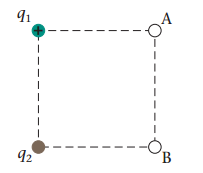# Problem: In (Figure 1), q1 =+1.7nC and q2 =−3.3nC, and the side length of the square is 0.12 m. Part A. What is the electric potential at point A? Part B. What is the electric potential at point B?

###### FREE Expert Solution

The electric potential at a point is:

$\overline{){\mathbf{V}}{\mathbf{=}}\frac{\mathbf{kq}}{\mathbf{r}}}$

95% (112 ratings)###### Problem Details

In (Figure 1), q=+1.7nC and q=−3.3nC, and the side length of the square is 0.12 m.Part A. What is the electric potential at point A?

Part B. What is the electric potential at point B?

Frequently Asked Questions

What scientific concept do you need to know in order to solve this problem?

Our tutors have indicated that to solve this problem you will need to apply the Electric Potential concept. You can view video lessons to learn Electric Potential. Or if you need more Electric Potential practice, you can also practice Electric Potential practice problems.